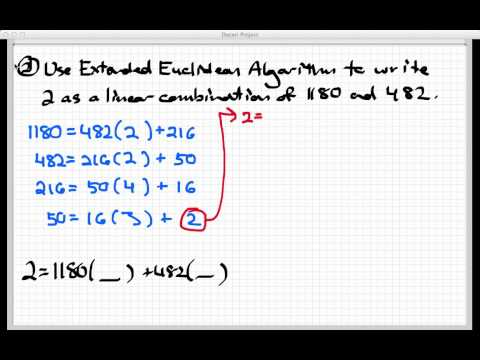#### Euclid Algorithm and Extended Euclid Algorithm Tutorial - 1

Created by inventionsbyhamidinventionsbyhamid

inventionsbyhamid,

Note: This tutorial is still in development

##### Euclid's Algorithm

Euclid Algorithm is used for finding Greatest Common Divisor(GCD) of two numbers. GCD of two numbers is the largest number that divides both of the numbers. Example GCD(10,16) = 2 . The common method we use for finding GCD of two numbers manually is the Euclid's algorithm where we divide the larger number by smaller number and the remainder is then made the divisor and the smaller number as dividend. This process is repeated until remainder becomes 0. The last divisor is the required GCD. Following example will make the process clear :
To find GCD(10,16)

Divisor Dividend Remainder
10 16 6
6 10 4
4 6 2
2 4 0
GCD(10,16) = 2

Implementation Code

``````int gcd(int a, int b) {

if (b==0) return a;

return gcd(b,a%b);
}
``````

Time Complexity : O(log(max(A, B)))

##### Extended Euclid's Algorithm

GCD(A,B) has a special property that it can always be represented in the form of an equation, i.e., Ax + By = GCD(A, B). Extended Euclid's Algorithm is used to find integer coefficients x and y in `Ax + By = gcd(A, B)` where A,B are known non-zero integers which is from Bézout's identity. The algorithm is useful in finding the modulo inverse and in solving linear congruences using Chinese Remainder Theorem. Before moving on to the code implementation here is a great video that explains how the algorithm worksJava Implementation Code

``````//returns array with ans=gcd, ans=X, ans=Y as elements for equation Ax+By=gcd
private static int[] extendedEuclid(int A,int B){
int ans[] = new int;
if(B==0){
ans = A; //gcd = A
ans = 1; //x = 1
ans = 0; //y = 0
}
else {
ans = extendedEuclid(B,A%B);
int temp = ans;//temp = x
ans = ans;//x = y
ans = temp - (A/B)*ans;//y = x – (A/B)*y
}
return ans;
}
``````

Time Complexity : O(log(max(A, B)))

##### How we came up with x = y and y = x – (A/B)*y ?
``````  Ax + By = gcd    (1)
if x1 and y1 are results for inputs A%B and B
B*x1 + (A%B)*y1 = gcd

When we substitute A%B = (A - (⌊A/B⌋)*B) i.e. Remainder = Dividend - Quotient * Divisor in above, we get following.

B*x1 + (A - (⌊A/B⌋)*B)*y1  = gcd   (2)
Note that ⌊a/b⌋ is floor(a/b)

Above equation (2) can also be written as below
A*y1 + B*(x1  - (⌊A/B⌋)*y1) = gcd    (3)

After comparing coefficients of 'A' and 'B' in (1) and (3), we get following

y = x1 - ⌊A/B⌋ * y1
x = y1
``````

Last updated